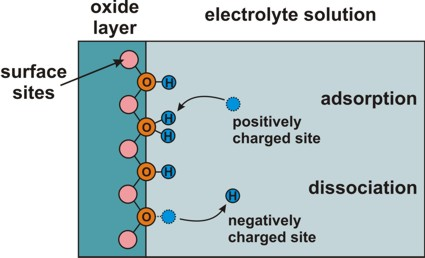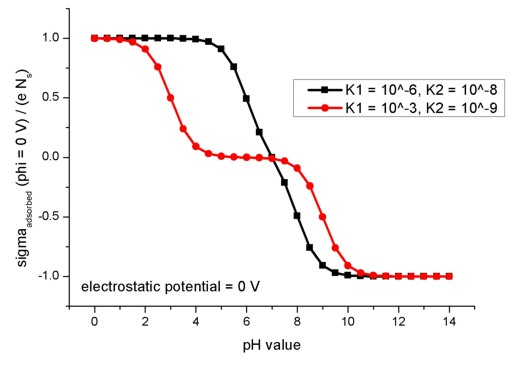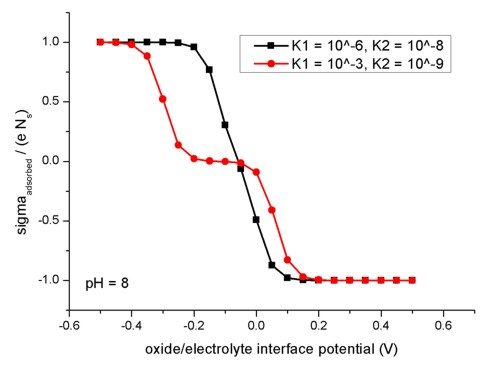nextnano.com  nextnano³  Download | Search | Copyright | Publications  * password protected nextnano³ softwareinterface-states

# interface-states

To specify additional charges at material interfaces, one has to specify
- material interfaces
- interface state properties.
See also documentation under keyword``` \$material-interfaces```.

```!-------------------------------------------------------------! \$interface-states                                   optional  !  state-number                       integer         required  !  state-type                         character       required  ! trap, fixed-charge, electrolyte  interface-density                  double          required  !  number-of-energy-levels            integer         optional  ! ```for``` trap  energy-levels-relative             double_array    optional  ! ```for``` trap  degeneracy-of-energy-levels        integer_array   optional  ! ```for``` trap  transition-times-cb-to-levels      double_array    optional  ! ```for``` trap  transition-times-levels-to-vb      double_array    optional  ! ```for``` trap                                                               !  adsorption-constant                double          optional  ! ```for``` electrolyte  dissociation-constant              double          optional  ! ```for``` electrolyte                                                               !  pressure                           double          optional  ! ```for``` gas  surface-phonon-frequencies         double_array    optional  ! ```for` gas `(1st = weakly, 2nd = strongly chemisorbed surface state)```  accomodation-coefficients          double_array    optional  ! ```for` gas `(1st = weakly, 2nd = strongly chemisorbed surface state)```  energy-levels-chemisorbed-states   double_array    optional  ! ```for` gas `(1st = weakly, 2nd = strongly chemisorbed surface state)```  free-molecule-energy               double          optional  ! ```for``` gas  molecule-mass                      double          optional  ! ```for``` gas                                                               ! ``````\$end_interface-states                               optional  ! !-------------------------------------------------------------!```

## Syntax

```state-number                  = 1                               = 2                               = integer ```Refers to` state-numbers `specified in``` \$material-interfaces```.

```state-type                    = fixed-charge                               = trap            ! ```The trap model is not fully tested yet. We don't have any tutorials for it.```                               = electrolyte                               = gas  ```

• `fixed-charge`

```interface-density         = -2.2d13   ! -2.2 x 1013 [|e|/cm2] ```interface density of``` fixed-charge ```in units of``` [e/cm2]  ```
• `trap```` interface-density         = 1.0d15    ! 1.0 x 1015 [1/cm2] ```interface density of impurity type in units of``` [1/cm2] number-of-energy-levels   = 1 ```number of energy levels of this impurity

```energy-levels-relative    = 0.3d0 ! ```in units of``` [eV] ```(can be an array of energy levels```) ```energy levels in [eV] relative to 'nearest' band edge (n-type `->` conduction band, else valence band)

```degeneracy-of-energy-levels = 2 ! ``` for donors```                             = 4 ! ```for acceptors```                                ``` ``` ! ```can be an array of degeneracies (one for each energy level)``` ``` degeneracy of energy levels

```transition-times-cb-to-levels =  ! ```can be an array of transition times``` ```required in case of``` trap```: times from conduction band to discrete levels
``` transition-times-levels-to-vb =  ! ```can be an array of transition times``` ```required in case of``` trap```: times from discrete levels to valence bands

Not included yet:
-` relevant_bandedgeV = 1: `Ionization energy relative to band edge of left octant
-` relevant_bandedgeV = 2: `Ionization energy relative to band edge of right octant

• `electrolyte`

Definition of electrolyte: An aqueous solution containing dissolved ions that result from the dissociation of salts.
The surface ionization that occurs at the oxide/electrolyte interface yields an interfacial sheet charge density.
(Note: The pH value is specified in the keyword``` \$electrolyte```.)

There are two ways how the electrolyte influences the calculations:
- oxide/electrolyte interface states:```             \$interface-states```
- Poisson-Boltzmann equation in electrolyte region:``` \$electrolyte                                       \$electrolyte-ion-content```

```!--------------------------------------------------------------------! ! Ga(x)O(y) behaves similarly to Al2O3 surface: 8.0d14 = Al2O3 value ! !--------------------------------------------------------------------! ! Amphoteric surface !--------------------------------------------------------------------! ! S: oxide molecular site with a bonded hydroxyl group OH ! ! Two surface reactions: !  SOH_2^+ <=> SOH  + H^+ : dissociation constant K_1 = adsorption-constant !  SOH     <=> SO^- + H^+ : dissociation constant K_2 = dissociation-constant ! ! SOH    : neutral ! SOH_2^+: positive ! SO^-   : negative ! ! total density of surface sites = total number of surface sites per unit area = n_s ! n_s = nu_'SOH' + nu_'SOH_2^+' + nu_'SO^-' !--------------------------------------------------------------------! ```
Electrolyte: Site-binding model (interface charges)
`  => `semiconductor/electrolyte or oxide/electrolyte interface
`  => `Amphoteric behavior of surface: Adsorption or dissociation of hydrogen ions at hydroxyl (OH) groups.``` ```          These two reactions are characterized by two dissociation constants K1 and K2.
`     `Adsorption and dissociation at this interface leads to an interface charge.``` interface-density         = 8.0d14   ! 8.0 x 1014 [1/cm2]```interface density of surface sites Ns in units of` [1/cm2]`total density of surface sites, e.g. 'surface hydroxyl groups```' (S-OH) adsorption-constant       = 1.0d-8  ! K1 = adsorption   constantdissociation-constant     = 1.0d-6  ! K2 = dissociation constant```These refer to the chemical reactions at the surface of the semiconductor (or oxide) that are due to the presence of the electrolyte.
These constants are material parameters of the semiconductor (or oxide).
In units of` [-]`.``` ```More information on the electrolyte liquid and the Poisson-Boltzmann equation:``` \$electrolyte                                                           \$electrolyte-ion-content```

The following figure shows the relation of the oxide/electrolyte interface charge density sigmaadsorbed divided by the maximum possible oxide/electrolyte interface charge density e Ns for different pH values. Here, the electrostatic potential is taken to be fixed at phi = 0 V. The model used here applies to amphoteric surfaces. For details confer Fig. 2.2.3 and the related description in the diploma thesis of Michael Bayer, TU Munich (2004).The figure shows the results for two different combinations of absorption and dissociation constants.

```   adsorption-constant   = 1d-6    ! K1 = adsorption   constant    dissociation-constant = 1d-8    ! K2 = dissociation constant    adsorption-constant   = 1d-3    ! K1 = adsorption   constant    dissociation-constant = 1d-9    ! K2 = dissociation constant```

The following figure shows the relation of the oxide/electrolyte interface charge density sigmaadsorbed divided by the maximum possible oxide/electrolyte interface charge density e Ns for different oxide/electrolyte interface potential values. Here, the pH value is taken to be fixed at pH = 8. The model used here applies to amphoteric surfaces. For details confer Fig. 2.2.4 and the related description in the diploma thesis of Michael Bayer, TU Munich (2004).To create this figure, we applied``` flow-scheme = 31```.

• `gas`:

The gas model is based on the so-called Wolkenstein model (Volkenstein) which is a charge transfer model (and which is an improvement with respect to S.R. Morrison's classical "charge transfer model").
It consists of a weakly and a strongly chemisorbed surface state.
Related terms: Electroadsorptive effect, Wolkenstein isotherm

`- `Advanced Gas Sensing: The Electroadsorptive Effect and Related Techniques
`  `T. Doll (Ed.)
`  `Kluwer Academic Publishers, Boston, 2003, ISBN 1-4020-7433-6

`- `Chemisorption effects on the thin-film conductivity
`  `H. Geistlinger```   ```Surface Science 277, 429 (1992)

```!interface-density                = ```
```0d0             ! ```no gas-interface model``` interface-density                = ``````1d12            ! [cm-2] ```total density of surface sites```!pressure                         = 50d0            ! [Pa]  50  Pa = 50  N/m````2```` ```(low  O2)``` pressure                         = ``````20d3            ! ````[Pa]  ```` 20 kPa = 20 kN/m2 ```(high O2)```  surface-phonon-frequencies       = ``````1d13    1d13    ! ````[Hz] `v0, v`-````   1 * 1013 Hz = 1 * 1013 1/s                                                    ! ```(1st = weakly, 2nd = strongly chemisorbed surface state)```                                                    ! ```vibration frequency of the adsorbed particle (typical value: ~1013 Hz)```  accomodation-coefficients        = ````1d0 ` ```    1d0     ! [] ```alpha0, alpha`-`
```                                                    ! ```(1st = weakly, 2nd = strongly chemisorbed surface state)```                                                    ! ```alpha = accomodation coefficient```  energy-levels-chemisorbed-states = ```
```-3.80d0 -7.90d0 ! ```Ea0, Ea`-````  [eV]                                                    ! ```(1st = weakly, 2nd = strongly chemisorbed surface state)```  free-molecule-energy             = ``````-3.60d0         ! [eV] ```(Comment: Is this property related to electron affinity?)``` molecule-mass                    = ``````31.9988d0       ! [u]``````                                                    ! ```oxygen atom       O : mass of an atom     =      15.9994 u```                                                    ! ```oxygen molecule O2: mass of a molecule = 2 * 15.9994 u = 31.9988 u
```                                                    ! ```1 [u] = 1 / NA [g] = 1 / (1000 * NA) [kg], where NA is Avogadro's number.```  ```